main page   list of papers   library

Aethereal Breathing
Dedicated to the bright memory of my daughter Anastasia

True knowledge is a knowledge by causes.
Fransis Bacon

Taking as a fact  the presence of the ether in the Universe, united quasiisotropic and elastic medium, being the main basic matter, the carrier of the whole energy, all processes, occurring in the Universe, and taking for the base of beliefs about it working model [2-3], presenting it in the manner of twocomponent medium - corpuscular and phase, we will consider its some interesting effects caused by motion of celestial bodies within corpuscular ether, and moving of flows of phase ether in outer space.

At first we will do the little background for one, who did not read the previous articles about ether [2,3]. If you have read it, you can skip following paragraph.

#### The Ether States

According to working model  the ether consists of identical densepacked incondensable massless elements - "amers", interactions between which are spreading with greatly possible for this medium velocity - velocity of light. In the main ether, which we call corpuscular, there are separate empty cells - vacancies, which are forming the phase ether.

The corpuscular ether has a properties of pseudo-solid body with dodecahedron anisotropy in exclusive cases of impoverishing it by phase ether. The usual condition of corpuscular ether is pseudo-liquid.

The phase ether is pseudo-gas, having many properties of ideal gas.

Under the certained conditions a moving the particles of these two types forms the substance and the field. The Field is shift and rotation ether vibrations. The substance is a joint motion of ether corpuscles, having a mass property .

Motion of all substance particles occur in corpuscular ether, which is an absolute reference system for them. Herewith the wave phenomenas are appearing, reference system for which is a field of vacancies - the phase ether.

The Vacancies of light velocity, being carriers essential gravity puls we will name gravitons. That puls equals

Ig = 861 c2 / h [kg-1 s-1],

here h is Planck constant,
861 is full cycle of gravitons emission in a convoluted EM-wave (see ),
c - light velocity in corpuscular ether.

All bodies, having mass at rest, emit gravitons spherically symmetrical, generating around itself gravity field, which is a carrier of gravitational power. The last is measure of reduction of gravitational potential (density) of corpuscular ether. At the same time with that there is inverse flow of low-speed vacancies towards gravitating body. Thereby balance of flows or "law of conservation of amount of vacancies within the ether" exists.

Far from gravitating body, being carrier of whole energy of Universe, ether already inheres in condition of isotropic compression that is expressed in presence its maximum fixed potential:

 φo = co2[m2 s-2], (1)

here с0 - is a velocity of waves within empty ether, far from gravitating masses.

At velocities to much smaller than light velocity and on distances much smaller than cosmological, potential of ether compression in presence of gravitating bodies is described by known scalar field in differential equation of Poisson: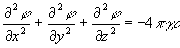[1/s2], (2)

here γ - is gravity constant [m3/(kg s2)],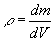- is volume density of mass distribution [kg/m3].

The Gradient of potential defines the gravity field tension (free fall acceleration) in given point of space: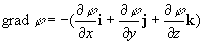[m/s2], (3)

here i, j, k - are orths of three-dimensional space.

Decision of equation (2) is: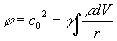[m2/s2], (4)

here r - is distance from measured points to gravitating volume dV.

Thence the law of universal gravitation is derivated by known way, diversified from classical by only cosmological adjustment, taking into account relaxation of ethereal vacancies: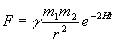[kg m/s2], (5)

here F - is gravity force,
γ - is gravity constant [m3/(kg s2)],
m1 , m2 - is gravitating masses [kg],
r - is distance between bodies [m],
H - is Hubble constant [1/s],
t - is a light distance between gravitating bodies [s].

The exponential multiplier in (5), defining by fading in the ether, which causes a braking of gravitons, excludes Zeeliger gravity paradox, providing exception influence of infinite remote masses.

As it is stated experimental, amount of vacancies in outer space practically constant. Besides outgoing flow there is inverse coming flow of vacancies to bodies exists, on direction of gradient of potential of the ether (3). This flow having velocity of order second cosmic, since follows gradient of potential, possesses measly pulse, practically not affecting on gravity, but affecting on precise measuring equipment

Iv = 861V2 / h [ kg-1 s-1 ],

here V - is velocity of vacancies, at the average equals second cosmic velocity for local gravity pits.

This value is an order of 10-8 graviton's pulse, since velocity of flows of phase ether reaches in vicinities of Earth values +20… - 40 km/s only.

Whereas, the balance of flows of vacancies and gravitons exists near gavitating bodies, in spite of low velocity of vacancies, so above their density, and above general their influence upon gravity field. So the general influence of flow of vacancies near gravitating bodies reaches the values of order 10-4 of gravity field.

Gravity Field in Corpuscular Ether

Notwithstanding idle fictions, not bodies influence each to another directly in space, but only gravity field of the ether, already residing in point of location of body, acts upon it. This logically follows from that it is no mechanism of "distant action" or snap action one bodies on another. Lets consider a mechanism of gravitation in detail.

The Body, having a mass radiates gravitons in all sides, as this is shown on fig. 1a. If in considered limits of this field there is no other body then with increase of distance density of gravitons decreases in square (each sectoral volume on drawing has one and ditto amount of gravitons).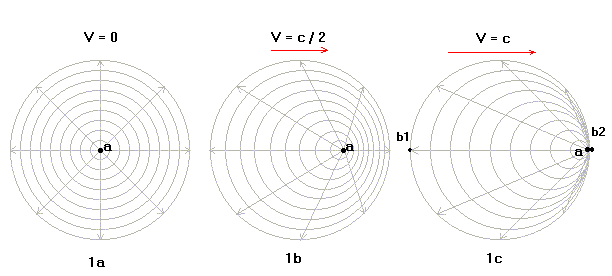Fig. 1. Distribution of gravity field of spherical body "a" under different velocities of its motion in corpuscular ether.

In any time field already portioned in space and "test" body, coming into this field follows its gradient and available beside it mechanical energy - kinetic and potential. That is to say its path is defined by the vector of kinetic pulse and distance from source of gravitation in any time.

What's occurs when moving the source of gravitation in corpuscular ether at the speed, commensurable with light velocity? The case of velocity of source to equal half of light speed is shown on fig. 1b, but equals to velocity of light is shown on fig. 1c.

The anisotropy of distribution of gravitons density (gravity field) appears with compaction that field on move of path of source and reduction of density in inverse direction. Moreover this anisotropy has such asymmetry that for "test" bodies b1, residing behind moving the source of gravitation of light velocity (see fig. 1c), density of gravity field will be in two times lower,in steady-state variant, but for body b2, residing on paths of move it is infinitely big at moment of collision with source of gravitation (and zero - before this moment).

Thereby speed anisotropy of gravity field exists in the manner of apparenting change the mass of gravity source on "standpoint" test body

 mapp = ms / (1-V/c) [kg] (6)

here mapp - is appearing mass of gravitating body;
ms - is true mass of gravitating body.
V - is absolute velocity of gravitating body in corpuscular ether
("+" for approaching body and "-" for move off);
c - light velocity.

If we consider joint motion of two bodies and accordingly their joint gravity field, that we will see that maximum detour from Newton's formula (5) will be in the case of their mutual location on axis of their motion within corpuscular ether. In that case this formula will look so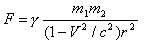[kg m s-2], (7)

In the case of non-alignment location of bodies effect of detour from Newton's formula will accordingly less. The Velocity of Earth moving in corpuscular ether reaches the value of order 10-3 of light velocity so deflection in formula (7) differs from Newton's formula not more than 10-6. This value is exactly appearing deviation of gravity constant, measured by means of symmetrical Cavendish's torsion balance, hanging from location of horizontal axis of that balance for motion within corpuscular ether. We will notice that here we do not speak about of flows of phase ether, influence of which we will consider below.

In the case of asymmetrical heavy body, carried to Cavendish's balance we have other dependency. There is a measuring of gravity field only of a single heavy side by measurements of elastic power of suspension twisting: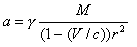[m s-2],

here M - is a mass of heavy body.

Thereby, appearing mass deviation (or appearing deviation of Newton's constant) will reach the value of order 10-3. Naturally that is no real change Earth's mass, nor gravity constant in considered cases. All these effects appearing only, as appearing small size of remoted objects on photo.

Gravitational Pits. Types of Mechanical Energy.

All physical experiments, carried out during history of science, prove that law of conservation of energy is kept highly strictly. There is only transformation of one type of energy into another under certain favouring that conditions. Due to that all diversity of world exists.

Lets consider transformation of energy of body under its drop in gravitational pit, that is to say into area of action of celestial body's gravitation, described by potential (4).

What's happen with its physical parameters?

1. The motion is speeding up to account of action of gradient (3) of gravity field. Herewith increases the kinetic energy of body, which is always positive by determination.

dWk = m g dS= m (dV/dt) Vdt = m VdV ; Wk = mV2/2 [kg m2 s-2].

2. Always negative by determination, potential energy of body is decreasing, on a value equals to growth of kinetic energy

dWp = - m g dH = - m (dV/dt) Vdt = - m VdV ; Wp = - mgH = - mV2/2 [kg m2 s-2].

3. Unless occurs the collisions of body with other bodies, not important macroscopic or microscopic, as well as radiations, that as body fell into gravitational pit, so it will leave that pit. In that case the orbit of body is hyperbolic.

4. If during process of body's moving across area of gravitational pit will occur dissipation of energy, that its hit into that pit will become inconvertible. Depending on value of dissipative energy a body will turn out to be on one or another potential level of gravitational pit, having become an element of this gravitational systems. This potential level will be strictly fixed at the absence of dissipation, fall at presence of it and increase in rare antientropical cases. Its spatial position unlike gravitational potential will have constant elliptic orbit according to the known laws celestial mechanics, changing only under influence of usually small dissipation. Thereby it saves total machanical energy

 Wm = Wp + Wk = const. [kg m2 s-2]. (8)

So even though celestial body inheres in aphelion i.e. at remoted point of orbit, it is all the same on same potential level gravitational pit. The difference is in instant correlation of kinetic and potential energy only. Only the bodies having other gravitational potential (4) inhere below or above of it in potential pit. Practically, mechanical energy of celestial body is zero outside of gravitation and product of its mass and always negative, if its measure from out of gravitation Space, gravitational potential:

Wm = mV2II / 2 = mVI 2

According to our working model, potential energy is always negative on determination, since it is defined by body fall into potential pit from undoubtedly highest gravitational potential c2.

The kinetic energy is always positive on determination. In absence of gravitation it is Wm = 0. In potential pit, when part of kinetic energy was dissipated Wm < 0. By dividing (8) on mass m, we will get:

Wm / m = V2 / 2 + gH = φ - φo = -VII2

Where is Kept the Potential Energy?

Notwithstanding of idle fiction the Space can not be a carrier of this energy, since it is indifferent to respect of concrete body. Logistical expect that carrier of this energy is body itself, interacting with potential gravitational field. However carrier of potential energy is not a kinetic energy of atoms of this body, but something other. To understand this lets consider unlucky case with lift in skyscraper. At lifting of booth of lift on height 425 metres a person by mass 72 kg gains the potential energy Wp = 72 kg + 425 m + 9.8 m/s2 =300000[kg m2/s2]. This energy enough for functioning one-kilowatt boiler for 5 minutes or heating of body of person more, than on 10C. However, as is well known, temperature of body i.e. kinetic energy of atoms of lifting body does not change.

However this energy doesn't kept somewhere, but within a body. What is a carrier of this energy? According to our working model of the ether, only ether itself is that carier.

Within the framework of our ether working model it is possible to expect (and experiments that confirm) that in process of change the position of body in gravity field the concentration of phase ether is changing, being kept in bodies pro rata loss / aquisition of amount of motion by body, thereby that specific concentration of phase ether to unit of mass is proportional to square root of local gravitational potential.

The Field of Low-speed Vacancies within Corpuscular Ether

The phase ether is at all other, different from gravity field phenomena. It is a field of low-speed vacancies. According to working model [2,3] distribution of low-speed vacancies, form as a result of deceleration of gravitons on cosmological distances and "dumping" in potential pits of gravity field of masses, has an original nature, particularities of which we will consider below.

The Amount of low-speed vacancies, seized by gravity pit naturally depends on its size. In usual starry-planetary case of central gravitating body, an intensity of flow of vacancies will be defined by degenerative kind of equation of Poisson (2)

 ∆(φ) = g / R = VII2 / 2R2 = VI2 / R2 [1/s2], (9)

here ∆ - is operator of Laplas (laplasian);
g - is gradient of gravity field (free fall acceleration);
VI - is the First Space Velocity;
VII - is the Second Space Velocity;
R - is distance from gravitating body.

According to working model of phase ether [2,3] its density with big accuracy corresponds to mass dencity of body with the exclusion of small deviations, in accordance with variations of flow (9), supplying the process of gravitons radiation by substance.

The Phase Ether within the Substance

According to brought above motives of our working model a substance is capacity of phase ether, amount of which in substance is

 Kpe = Nkg mVII , [vac] (10)

here Nkg - is a specific amount of phase ether in 1 kg mass [vac/kg] ;
m - is a mass of substance [kg] ;
VII - is the Second Space Velocity (square root of potential of local gravitational pit) [m/s].

Effects of Moving the Phase Ether

Any body not even having own mass, moving in fluid or pseudo-fluid medium such corpuscular ether, has so-called joined mass . Due to that all objects, having physical volume and impervious for this medium, has the inertia property. Accordingly that, the phase ether, when speed up motion is arising, must abandon its "home place".

Lets consider the main types of such motions and effects produced by itself.

Linear Accelerated Motion and Nikitin's Phenomena 2

Linear accelerated motion is defined by derivative of linear velocity

a = dV/dt [m s-2]

here V - is linear velocity [m s-1];

t - is time [s].

In the case of accelerated motion, the phase ether, which kept in usual physical body, "lags behind" from body and creates speed flow of phase ether in surrounding space, for instance, air or vacuum, striving justify the pressure of this pseudo-gas. Under big difference of density of body and surrounding medium even under usual values of speedups of several [m/s2], the flow of phase ether, having velocities of hundreds [km/s], appears in body surrounding medium. It is easy to find it by deflection of ray of light, coming from accelerated body. As I know, this effect is discovered by George Nikitin.

Rotary Motion and Searl's, Podkliotnov's, Roshin-Godin's Effects

Acceleration during rotary motion is defined by product of tangential linear velocity with angular velocity of rotation

a = Vω = V2/r [m s-2]

here ω = V/r - is angle velocity [s-1];
r - is radius of rotation [m].

Under given type of motion phase ether scatters radially from revolvinging body. However in usual conditions that brings only surrface flow of phase ether from equator to poles, generating many well known effects in geophysics, meteorologies, but not finding due causal explanation.

For instance, since phase ether causes vortices of corpuscular ether during its motion, which is nothing else than magnetic field, that appears magnetic field of the Earth, supported and intensified by Earth core.

In the case of special measures, undertaken (till now not by causes ;-) for delay of flow of phase ether, returning into body, concentration of phase ether inside body becomes anomaloused low. It brings that normal emission of gravitons becomes impossible, and gravitational mass of body decreases, pro rata reduction of concentration of phase ether in body.

How we can delay the flow of phase ether? The answer is simple: by magnetic field, which creates the obstacle to free moving the phase ether. It is possible to do it effectively by creation moving desksides of magnetic field in the manner of "blades", executing role of blades of vacuum pump turbine.

Planetary Motion and Effects of Izmailov-Karagioz-Parkhomov

During celestial bodies motion within garavitational pits, where exists distribution of phase ether, defined by equation of Poisson (2) and (9), density of phase ether in body is defined by fluctuations in process of orbital motion within gravitational pit and acceleration created by that pit.

g = VI2/R [m s-2]

here VI - is the First Space Velocity [ m s-1];
R - is distance between body and barycentre of gravitational pit [m].

Under given type of motion the absolute values of acceleration are measly, but effects generated by these speedup are enough significant to observe them. This is explained by the big values of mass, used in this case. The value of flows of phase ether and their variation highly significant. This reveals itself in origin of appearing variation of Newton's Gravitational Constant, measured by group of scientists (Izmailov V.P., Karagioz O.V., Parkhomov A.G. [5-10] ), indeed caused not by changing the masses of bodies, change the emission of gravitons, but in causes of variation of flows of phase ether. These researchers discovered the dependency of measured values of Gravitational Constant from starry day, being manifestation of other type of effects - shock waves, appearing during Earth motion in corpuscular ether.

Below we will consider the mechanism of these flows and physical effects, caused by its variation in detail.

Model of Low-speed Vacancies Flows in System of Gravitating Bodies

Variations of low-speed vacancies appear according to changings of gravitational field configuration in system of two or more celestial bodies.
For finding of these variations and calculation by its level absolute value of density of phase ether in substance [vacancies / kg] lets build the quantitative model of process of vacancies exchange by gravitational bodies. For clarity of models we shall use its electric analogue shown on figure 2.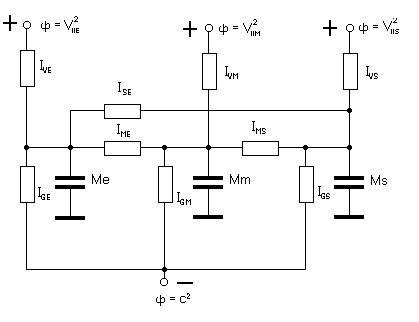Fig. 2. Electrical model of the process of exchange by low-speed vacancies in system of three celestial bodies Sun-Earth-Moon. (Igx - is flows of gravitons, Ixy - flows of phase ether exchange)

Positive sources of current in this model are low-speed flows of vacancies from interstellar Space. Negative sources are discharges of gravitons to interstellar Space. Capacitors are analogues of mass of celestial bodies. Currents between capacitors are analogues of low-speed vacancies flows, arising during change of configuration of gravitational parameters of bodies. Exactly that parameters can be the indicators of phase ether volume inside celestial bodies, which play a role of electric charge.

Like that, as in electrical engineering the electric capacity are measuring, applying a test alternating current on capacitor plates and determine the capacity from a value arisenned voltage, so and we will determine a charge of phase ether in celestial body through the known its capacity - mass and variations of phase ether flow caused by orbital motion, measured in experiment.

According to working model a pressure of flow of redistribution of low-speed vacancies must be proportional to force of influence on celestial body in gravitational pit

 F = m dV/dt = mV2/R [kg m s-2] (11)

here m - is mass of celestial body;
dV/dt - is its acceleration;
V - is velocity of body comparatively barycentre (lowest point of gravitational pit);
R - is distance between body and barycentre.

In turn, in connection with accumulation of phase ether by celestial body, flow, caused this pressure, must be an integral of pressure (11), throwing phase ether from gravitational pit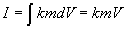[vac m/s],

here k - is density of phase ether (amount of vacancies in one kilogram [vac/kg] ),
that is to say it is proportional to variation of body's amount of motion inside gravitational pit.

On diagram fig. 2 curves of change corresponding to parameters for surface of Earth with provision for influences of orbital moving the Moon and gravities of Sun are shown.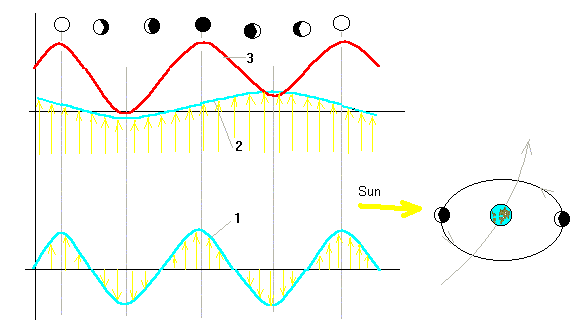Fig. 2. Diagram of change the parameters of gravitational mode of celestial body (Earth) in the system of three gravitating bodies with quasicircular orbits (1 - tangential component of variation of Earth motion from moving the double Earth-Moon system, 2 - radial component of variation of motion from barycentre of Solar system, 3 - total flow variation.).

Calculation of Flow of Gravitons, Produced by the Earth

For that at first we must calculate the intensity of production of gravitons by one electron

Nge = me Ige = me861c2 / h = 9.109 ·10-31 · 861· 9 ·1016 / (6.626 ·10-34) = 1.064·1023 [grav/s]

And now, by the Earth per second

NgE = Nge ME / me = 5.96 ·10 24 ·1.064·1023 / 9.109 ·10-31 = 6.962 ·1077 [grav/s] (12)

Thence by Earth for Moon synodial period

NgEM = NgE TM = 6.962 ·1077 · 29.53 · 86400 = 1.776 ·1084 [grav]

Calculation of Concentration of Phase Ether

From formula (9) it is possible to find relative value of fraudulent flows of phase ether between Earth and Moon, Earth and Sun in process of orbital moving the Earth-Moon system:

DEM = cos(2α) gM / RM = cos(2α) 3.319∙10-5 / 3,844∙108 = cos(2α) 8.634 ∙10-14 [s-2], (12)

DES = cos(α) gS / RS = cos(α) 5.903∙10-3 / 1,496∙1011 = cos(2α) 3.946 ∙10-14 [s-2], (13)

here α - is Moon orbital angle, counted out from vector of orbital motion
of Earth-Moon system;
g - are gravitational accelerations corresponding to celestial bodies;
R - are distances between celestial bodies.

Curves (12) and (13) and its sum are shown on fig. 2 and 3.

Knowing values (12) and (13), their correlation with constant flow of phase ether, spent by Earth on production of gravitons, as well as corresponding to change the mass of Earth in process of its orbital motion, it is possible to define the relative amounts and absolute amount of vacancies of phase ether, kept in 1 kilogram of mass.

For determination of defect of mass of the Earth, appearing in process of its motion we will calculate the amplitude of kinetic energy of Earth motion comparatively barycentre of Earth-Moon system. By the law of conservation of amount of motion, bodies velocities back proportional to its masses and amplitude of kinetic energy of tangential fluctuations of Earth (along orbit around Sun) equals to

Wka = ME(VM MM / ME)2/2 = (1.023 ·103·7.35 ·1022)2 / (2 · 5.96 ·1024) = 4.743 ·1026 [kg m2/s2]

here ME - is Earth mass; MM - Moon mass; VM - is Moon velocity.

Mass equivalent of this energy is

mk = Wka / c2 = 4.743 ·1026 / (2.998 ·108)2 = 5.28 ·109 [kg],

that is 5.28 ·109 / 5.96·10 24 = 8.854 ·10 -16 of Earth mass.

We know that between vacancies of phase ether and gravitons the balance of "conservation of gravitational charge" exists. It is revealled in experiments of G. Nikitin, and it is possible to confirm that amount of gravitons emitted by Earth for synodial period of Moon practically equals to absorbed amount of vacancies of phase ether. NgEM = NvEM.

The difference consists in not counting of annual orbital trend and influences of other planets of Solar system only.

As we know a concentration of phase ether in substance (10) and experimental correlation of velocities of flow of phase ether (12), caused by variation of kinetic parameters of Earth-Moon system to flow of gravitons, constantly emitted by Earth (refer to parameters processed by author and shown in table 1), it is possible to find amount of phase ether in 1 kilogram of substance:

Nkg =NgEMVEM / (md VII2) =

= 1.776·1084 · 8.634 / (5.2 · 5.28 ·10 9 ·1.1173 ·10 4 ) = 5.01 ·1070 [vac/kg]

Thence amount of vacancies, being kept in Earth is

NE = Nkg ME = 5.01 ·10 70 · 5.96·10 24 = 2,987 ·1095 [vac]

Thence and from formula (12) it is possible to get a time constant of consuption of vacancies by gravitating body

Tg = NE / NgE = 2.987 ·1095 / 6.962 ·1077 = 4.29 ·10 17 [s] = 13.6 ·109 [лет]

Thereby even though "to block" a flow of phase ether to body, it can provide emission of gravitons during a long time. Value of this fundamental constant, we will name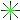ANASTASIA'S CONSTANT.
It equals to 1/H (Hubble Constant).

Validation of Working Model of Phase Ether Flows Motion

After data processing, got by George Nikitin, and removal in it two malfunctions of zero: -20" on April, 22-28 and -5" on May, 5-8 May we got following picture (fig. 3).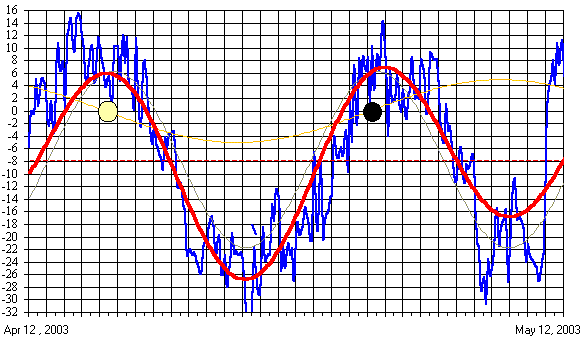Fig. 3. Corrected Nikitin's graph and Moon influence
on gravitational potential of Earth
(gray curve is accounting of tangential influence of Moon, yellow - is radial influence of Sun
on change the kinetic energy of Earth, red - is a joint influence of both factors)

On graph it is seen that curve of corner deviation with the exclusion of high-frequency noise with periods less a day, and, possible, annual trend, can be explained by variation of fraudulent flows of phase ether between Earth and Moon (gray curve), Earth and Sun (yellow curve), which total is shown by red curve.

The results of mathematical processing of these data are shown in table 1.

Table 1. Parameters of Flows of Phase Ether from Earth's surfaces

 parameter Earth Moon Sun angle magnitude [sec] -7.687 ±12.760 ±5.832 div grad Ф [s-2] 5.201 8.634 3.946 flow velocity [km s-1] VEE = -11.173 VEM = ±18.559 VES = ±8.482

That means that, either as follows from working model, phase ether is attracted and accumulated pro rata mass (density).

Near light velocity the corpuscular ether impoverished vacancies and begins "solidificate" that is to say gains anisotropic characteristics of six-axious dodecahedrical structure that highly important for explanation of substance characteristics .

As there was shown in  surplus concentration of phase ether during increasing the kinetic energy of planet well corellated with tectonic processes, which, as is well known, are motion of the great masses of Earth crust.

Gravitational Effects of Izmailov-Karagioz-Parkhomov

High-reliable average values of Newton's constant around 7 days beside new moon γ = 6.67332+0.000027 and 5 days following after full moon γ = 6.67179+0.000013 [5-10] are obtained by scientific group of The World Centre of Solid Earth. The Results their perennial precise measurements of gravitational constant are submitted on the site of this World centre. Perennial statistics of measurements brought about phases of synodial period of Moon is shown on figure 4. With the whole conspicuity these data carry the same nature, as given by G. Nikitin (fig. 3), including difference in minimums on old and young moon, corresponding to theoretical curve 3 on figure 2.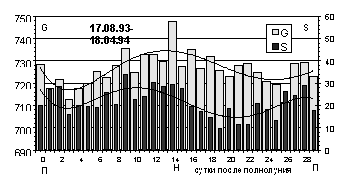Fig. 4. Deviation of measured values of Gravitational Constant
(two most significant digits of GC=6.6 are omitted)

Following to our working model of the ether in this case it is possible to select two components from discovered variations, which characterize tangential and radial gravitational components in the manner of corresponding to amplitudes of gravity fild variation, in accordance with flow of low-speed vacancies of phase ether

∆γt = ± 6.2 ·10-15 [m3 kg-1 s-2]; ∆γr = ± 3.0 ·10-15 [m3 kg-1 s-2].

During the Earth motion at the speed of 390+30 km/s across moveless corpuscular ether in spite of its superfluidity amust appear the phenomenas of type of shock wave in phase ether, "nib", which must be expressed in origin corresponding to compaction and rarefaction of phase ether.

The sectional view of the Earth, which has layers of different density, up to 10 [kg/dm3] is shown on figure 5. Here the distribution of "nibs" of shock waves are shown.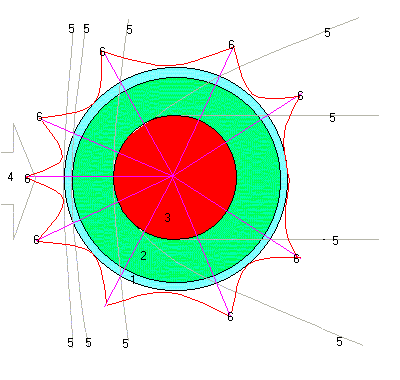Fig. 5. The Model of Earth Interaction with Corpuscular Ether.
(1 - crust 2.8 [kg/dm3], 2 - mantle 5.5 [kg/dm3], 3 - core 10 [kg/dm3], 4 - flow of corpuscular ether, 5 - shock waves of phase ether compaction, 6 - picks of phase ether distribution on the Earth surface).

Distribution to density of phase ether, built from experimental data , presenting variations of Gravitational Constant depending on starry day, is shown on figure 6.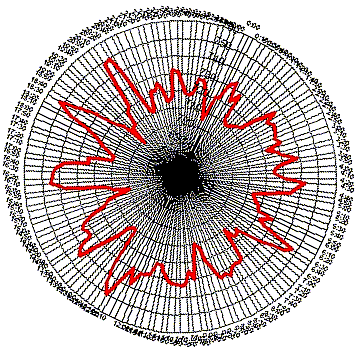Fig. 6. Experimental distribution of Gravitational Constant variations from time of starry day.

Consequences Following from Working Model of Phase Ether Flows

The phase ether is wholly discovered phenomenon of Nature. It behaves as a gas in many cases. Its presence, concentration and motion tells us about fine effects in gravity and electromagnetic fields.

Notwithstanding edle fictions, the bodies don't interact themselves in Space directly, but only through ether gravity field, being present in a place of location of body. This logically follows from absence of "distant action" mechanism or snap action one body to another.

The phase ether is accumulated inside substance pro rata density and square root of local gravity potential. Its flows define the potential energy of body.

All variations of Newton's Gravitational Constant are only appearing, connected with process of measurement itself in ethereal gravity field.

Changing the mass during motion occurs only to account of consuption of internal energy of body to kinetic energy. There are no other speed effects.

Hubble Constant is really universal constant, not hanging from anything. As it is shown in presented work, it can be found not from astronomical data, but by measuring of local physical characteristics of the ether.

References

1. Khaidarov K.A. Eternal Universe. - Borovoye, 2003.
2. Khaidarov K.A. Gravitating Ether. - Borovoye, 2003.
3. Khaidarov K.A. The New Lights. - Borovoye, 2003.
4. Joined Masses. Physical Encyclopedial Dictionary, p.587. - Moscow, Soviet Enc. Publishers, 1983.
5. Izmailov V.P., Karagioz O.V., Kuznetsov V.A., Melnikov V.N., Rosliakov A.E. Temporary and Spatial Variations of Measured Values of Gravitatonal Constant. Measuring technology, № 10, (1993), p.3-5.
6. Karagioz O.V., Izmailov V.P., Kuznetsov A.I. Strategy and Results of Studies of Ways of Increasing of Cavendish's Gravitational Constant Accuracy. High School News. Geodesy and Aerophotography, N 2, (1992), p.91-101.
7. Karagioz O.V., Izmailov V.P., Silin A.A., Dukhovski E.A. in book: Universal Gravity and Theories of Space and Time, (editor J. P. Terletski), M., UDN Publishers, (1987), p.102-126.
8. Karagioz O.V., Izmailov V.P., Parkhomov A.G. The Study of Fluctuation of Gravitation Constant Measurements on Torsion Balance Installation . Preprint N 21 MNTC VENT. M., (1992), 25 p.
9. Karagioz O.V., Izmailov V.P. The Measurement of Gravitation Constant by Torsion Balance. Measuring Technology, N 10, (1996), p.3-9.
10. Karagioz O.V., Izmailov V.P., Parkhomov A.G. The Study of Results of of Gravitational Constant Measurement. The Physical Thought of Russia. N 1/2 1999. p. 20-26.

Karim Khaidarov
Borovoye, November 1, 2003.
The Date of Registered Priority is November 1, 2003

main page   list of papers   library